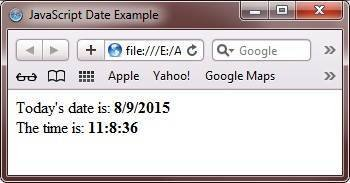# JavaScript Date

The Date object in JavaScript is used to display a date on a Web page.

## JavaScript Date Object Properties

The following table describes the properties of Date object in JavaScript.

Property Description
constructor holds the value of the constructor function that has created the object
prototype adds properties and methods to the Date object

## JavaScript Date Object Methods

The following table describes the methods of the Date object in JavaScript.

 getDate() returns the day of the month (ranges from 1 to 31) getDay() returns the numerical equivalence of the day of a week (ranges from 0 to 6). 0 for Monday getFullYear() returns the numerical equivalence of the year (in 4 digits) getHours() returns the hours (ranges from 0 to 23) getMilliseconds() returns the milliseconds (ranges from 0 to 999) getMinutes() returns minutes (ranges from 0 to 59) getMonth() returns the numerical equivalence of month (ranges from 0 to 11) getSeconds() returns the seconds (ranges from 0 to 59) getTime() returns the number of milliseconds since midnight Jan 1, 1970 getTimezoneOffset() returns the difference of time in minutes between GMT and the local time getUTCDate() returns the day of the month (ranges from 1 to 31) as per the universal time getUTCDay() returns the numerical equivalence of the day of the week (ranges from 0 to 6) as per the universal time getUTCFullYear() returns the year in four digits as per the universal time getUTCHours() returns the hour (ranges from 0 to 23) as per the universal time getUTCMilliseconds() returns the milliseconds (ranges from 0 to 9999) as per the universal time getUTCMinutes() returns the minutes (ranges from 0 to 59) as per the universal time getUTCMonth() returns the numerical equivalence of month (ranges from 1 to 31) as per the universal time getUTCSeconds() returns the seconds (ranges from 0 to 59) as per the universal time Parse() parses a date string and returns the number of millisecond since the midnight of January 1, 1970 setDate() sets the day of a month (ranges from 1 to 31) setFullYear() sets the year in four digits setHours() sets the hours (ranges from 0 to 23) setMilliseconds() sets the milliseconds (ranges from 0 to 999) setMinutes() sets the minutes (ranges from 0 to 59) setMonth() sets the numerical equivalence of month (ranges from 0 to 11) setSeconds() sets the seconds (ranges 0 from 59) setTime() sets a date and time by adding or subtracting specified milliseconds to/from midnight January 1, 1970 setUTCDate() sets the day of the month (ranges from 1 to 1) as per the universal time setUTCFullYear() sets the year in four digits as per the universal time setUTCHours() sets the hours (ranges from 0 to 23) as per the universal time setUTCMilliseconds() sets the milliseconds (ranges from 0 to 999) setUTCMinutes() sets the minutes (ranges from 0 to 59) as per the universal time setUTCMonth() sets the numerical equivalence of month (ranges from 0 to 11) as per universal time setUTCSeconds() sets the seconds (ranges from 0 to 59) as per the universal time toDateString() converts the date into a string toLocalDateString() converts the date into a string as per local conventions toLocalTimeString() converts the time into a string as per local convention toLocalString() converts the Date object into a string as per local convention toString() converts the Date object into a string toTimeString() converts time into a string toUTCString() converts the Date object into a string as per the universal time UTC() holds the number of millisecond since the midnight of January 1, 1970, as per the universal time valueOf() returns the primitive value of the Date object

## JavaScript Date Example

Here is an example of JavaScript date. This JavaScript program will print the today's date and the current time as output:

```<!DOCTYPE html>
<html>
<head>
<title>JavaScript Date Example</title>
</head>
<body>

<script type="text/javascript">
var today_date_var = new Date();
document.write("Today's date is: <b>" + today_date_var.getDate() + "/" + (today_date_var.getMonth()+1) + "/" + today_date_var.getFullYear() + "</b><br/>");
document.write("The time is: <b>" + today_date_var.getHours() + ":" + today_date_var.getMinutes() + ":" + today_date_var.getSeconds() + "</b><br/>");
</script>

</body>
</html>```

Here is the output produced by the above JavaScript date example program:Here is the live demo output produced by the above Date object example in JavaScript. You will watch today's date and current time as output.

Tools
Calculator

Quick Links
Signup - Login - Give Online Test